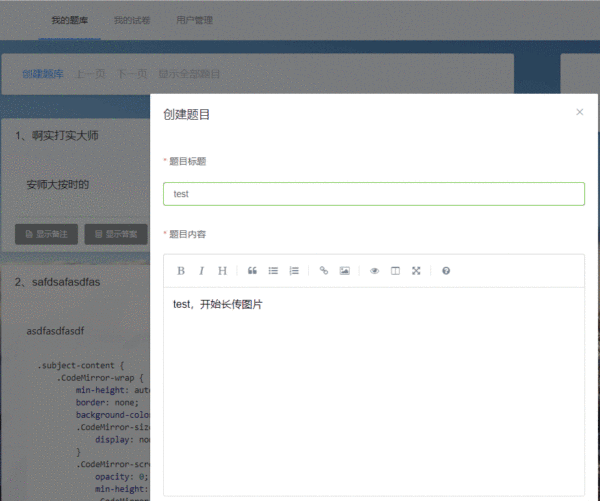# 拖拽

``````window.addEventListener("drop", e => {
e = e || event
if (e.target.className === 'CodeMirror-scroll') { // 如果进入到编辑器的话，将阻止默认事件
e.preventDefault()
}
}, false)
``````

`CodeMirror-scroll`这个Class就是`simplemde`编辑框的Class名称。

``````// 假设页面一共有三个编辑窗口，所以需要循环监听事件
[ this.\$refs.simplemde1,
this.\$refs.simplemde2,
this.\$refs.simplemde3
].map(({simplemde}) => {
simplemde.codemirror.on('drop', (editor, e) => {
if (!(e.dataTransfer && e.dataTransfer.files)) {
// 弹窗说明，此浏览器不支持此操作
return
}

let dataList = e.dataTransfer.files
let imageFiles = [] // 要上传的文件实例数组

// 循环，是因为可能会同时拖动几个图片文件
for (let i = 0; i < dataList.length; i++) {
// 如果不是图片，则弹窗警告 仅支持拖拽图片文件
if (dataList[i].type.indexOf('image') === -1) {
// 下面的continue，作用是，如果用户同时拖动2个图片和一个文档，那么文档不给于上传，图片照常上传。
continue
}
imageFiles.push(dataList[i])  // 先把当前的文件push进数组里，等for循环结束之后，统一上传。
}
// simplemde.codemirror的作用是用于区分当前的图片上传是处于哪个编辑框
// 因为已经有了下面这段代码，所以上面的屏蔽默认事件代码就不用写了
e.preventDefault()
})
})
``````

``````[ this.\$refs.simplemde1,
this.\$refs.simplemde2,
this.\$refs.simplemde3
].map(({simplemde}) => {
simplemde.codemirror.on('drop', (editor, e) => {
if (!(e.dataTransfer && e.dataTransfer.files)) {
return
}
let dataList = e.dataTransfer.files
let imageFiles = []
for (let i = 0; i < dataList.length; i++) {
if (dataList[i].type.indexOf('image') === -1) {
continue
}
imageFiles.push(dataList[i])
}
e.preventDefault()
})
})
``````

# 粘贴

``````simplemde.codemirror.on('paste', (editor, e) => { // 粘贴图片的触发函数
if (!(e.clipboardData && e.clipboardData.items)) {
// 弹窗说明，此浏览器不支持此操作
return
}
try {
let dataList = e.clipboardData.items
if (dataList.kind === 'file' && dataList.getAsFile().type.indexOf('image') !== -1) {
}
} catch (e) {
// 弹窗说明，只能粘贴图片
}
})
``````

`dataList.getAsFile().type.indexOf('image') !== -1`这个句话是判断，粘贴的东西确认是图片，而不是其他东西。

`if`里的上传图片，不一样的地方是`[dataList.getAsFile()]`，因为为了统一格式，方便`uploadImagesFile`函数进行处理，我加上了`[]`，使之成为数组。`dataList.getAsFile()`就是获取文件实例了。

# 上传

``````uploadImagesFile (simplemde, files) {
// 把每个文件实例使用FormData进行包装一下，然后返回一个数组
let params = files.map(file => {
let param = new FormData()
param.append('file', file, file.name)
return param
})

let makeRequest = params => {
}
let requests = params.map(makeRequest)

return arr => {
return callback.apply(null, arr)
}
}

// 服务端返回的格式是{state: Boolean, data: String}
// state为false时，data就是返回的错误信息
// state为true时，data是图片上传后url地址，这个地址是针对网站的绝对路径。如下：
Promise.all(requests)
for (let i = 0; i < resps.length; i++) {
let {state, data} = resps[i].data
if (!state) {
// 弹窗显示data的错误信息
continue
}
let url = `![](\${location.origin + data})`  // 拼接成markdown语法
let content = simplemde.getValue()
simplemde.setValue(content + url + '\n')  // 和编辑框之前的内容进行拼接
}
}))
}
``````

``````Promise.all(requests)
// code
})
``````

``````axios.all(requests)
// code
})
``````

# 结尾# 参考 && 感谢

skecozo作者的laravel-demo项目里的部分代码

Lemon作者的《simplemde 实现拖拽、粘贴图片上传》文章

f-loat作者的vue-simplemde项目

wescossick作者的simplemde项目